# 从图(Graph)到图卷积(Graph Convolution)：漫谈图神经网络模型 (一)

• 本文的提纲与叙述要点主要参考了3篇图神经网络的Survey，分别是来自IEEE Fellow的A Comprehensive Survey on Graph Neural Networks 来自清华大学朱文武老师组的Deep Learning on Graphs: A Survey，以及来自清华大学孙茂松老师组的Graph Neural Networks: A Review of Methods and Applications，在这里向三篇Survey的作者表示敬意。
• 同时，本文关于部分图卷积神经网络的理解很多都是受到知乎问题高赞答案的启发，非常感谢他们的无私分享！
• 最后，本文还引用了一些来自互联网的生动形象的图片，在这里也向这些图片的作者表示感谢。本文中未注明出处的图片均为笔者制作，如需转载或引用请联系本人。

## 历史脉络

1. 图神经网络的概念最早在2005年提出。2009年Franco博士在其论文 中定义了图神经网络的理论基础，笔者呆会要讲的第一种图神经网络也是基于这篇论文。
2. 最早的GNN主要解决的还是如分子结构分类等严格意义上的图论问题。但实际上欧式空间(比如像图像 Image)或者是序列(比如像文本 Text)，许多常见场景也都可以转换成图(Graph)，然后就能使用图神经网络技术来建模。
3. 2009年后图神经网络也陆续有一些相关研究，但没有太大波澜。直到2013年，在图信号处理(Graph Signal Processing)的基础上，Bruna(这位是LeCun的学生)在文献 中首次提出图上的基于频域(Spectral-domain)和基于空域(Spatial-domain)的卷积神经网络。
4. 其后至今，学界提出了很多基于空域的图卷积方式，也有不少学者试图通过统一的框架将前人的工作统一起来。而基于频域的工作相对较少，只受到部分学者的青睐。
5. 值得一提的是，图神经网络与图表示学习(Represent Learning for Graph)的发展历程也惊人地相似。2014年，在word2vec 的启发下，Perozzi等人提出了DeepWalk ，开启了深度学习时代图表示学习的大门。更有趣的是，就在几乎一样的时间，Bordes等人提出了大名鼎鼎的TransE ，为知识图谱的分布式表示(Represent Learning for Knowledge Graph)奠定了基础。

## 图神经网络(Graph Neural Network)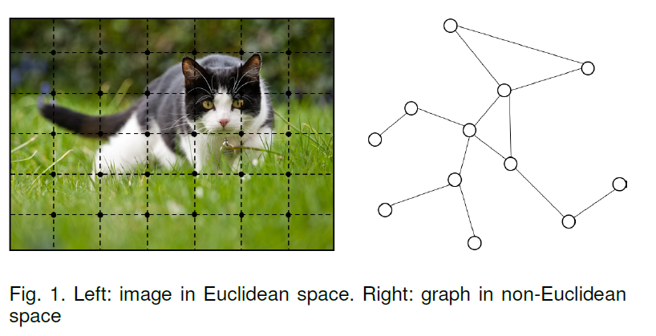### 状态更新与输出

$𝐡^{t+1}_𝑣=𝑓(𝐱_𝑣,𝐱_𝑐𝑜[𝑣],𝐡^{t}_𝑛𝑒[𝑣] ,𝐱_𝑛𝑒[𝑣]),$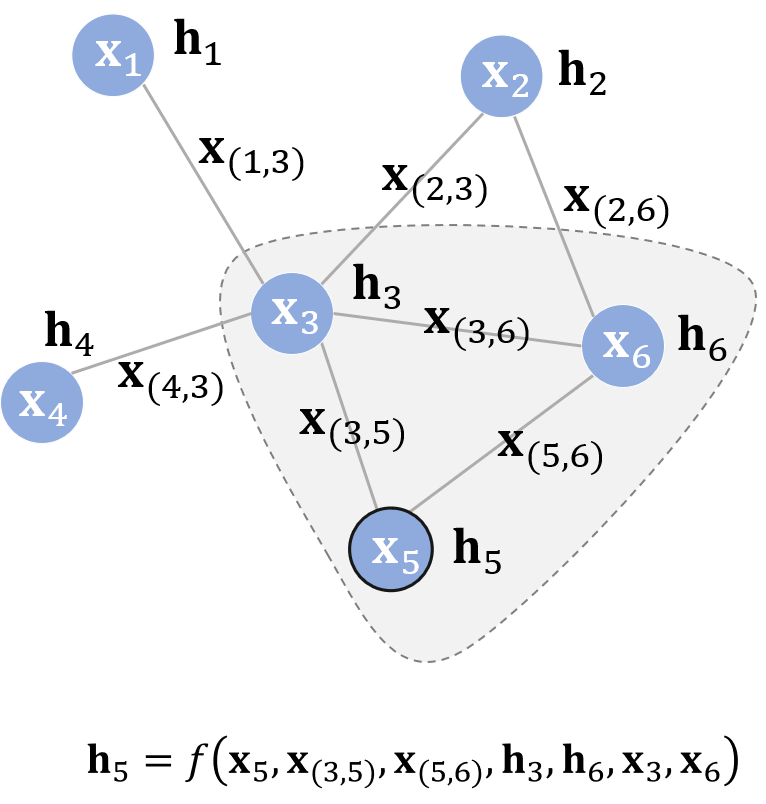$𝐨_𝑣=𝑔(𝐡_𝑣,𝐱_𝑣)$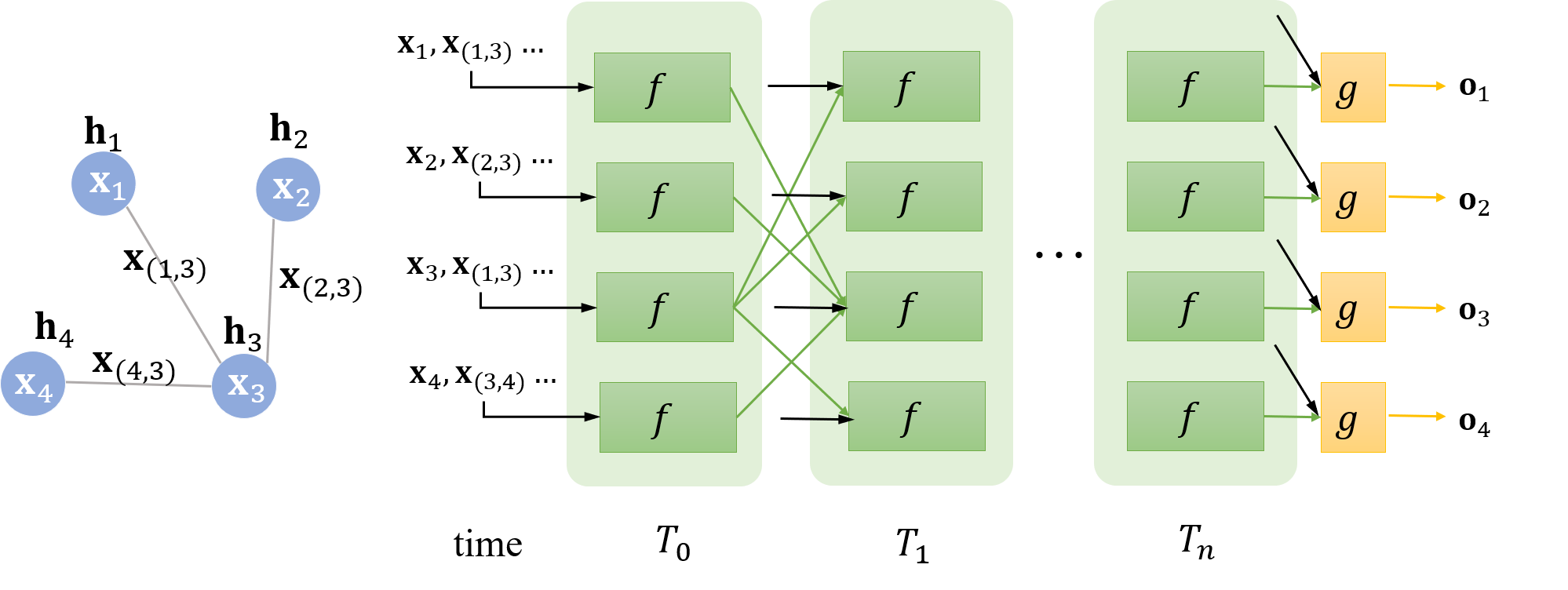$||\mathbf{H}^{t+1}||_{2}-||\mathbf{H}^{t}||_{2}<\epsilon$

### 实例:化合物分类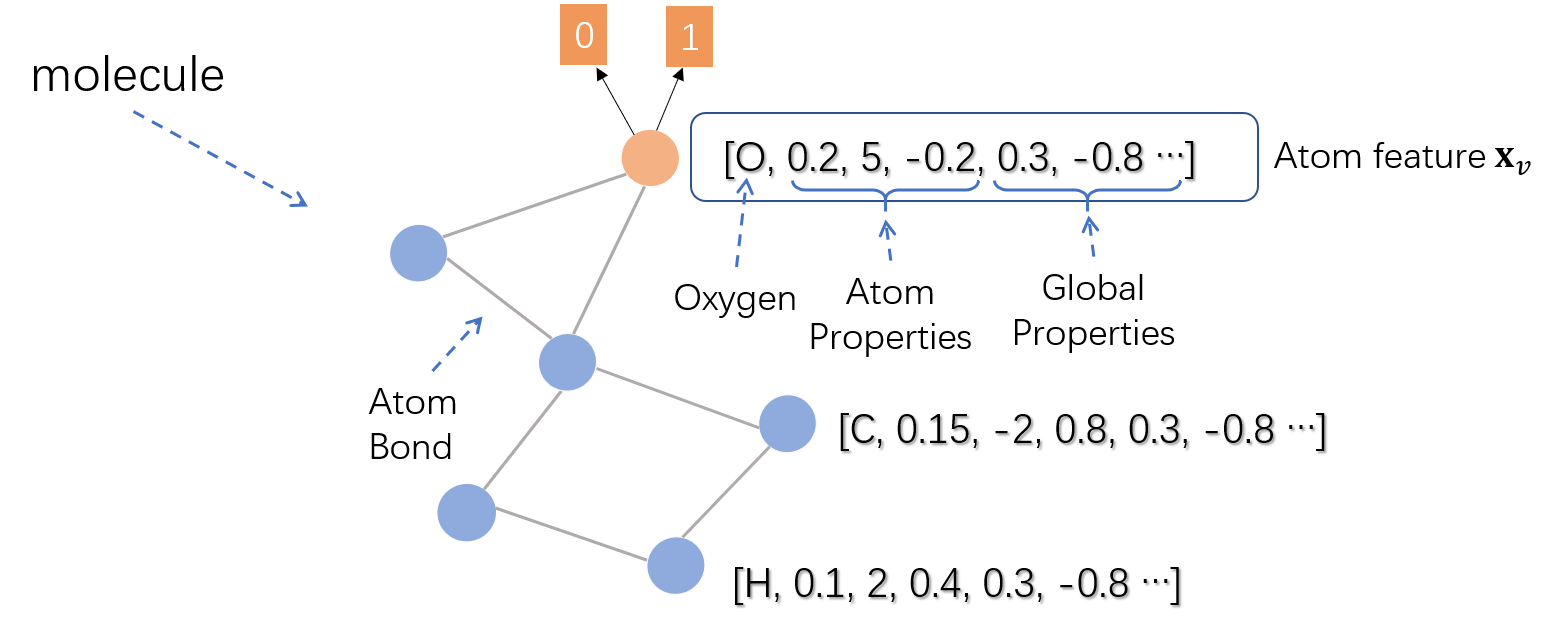### 不动点理论

$𝐇^{𝑡+1}=F(𝐇^𝑡,𝐗)$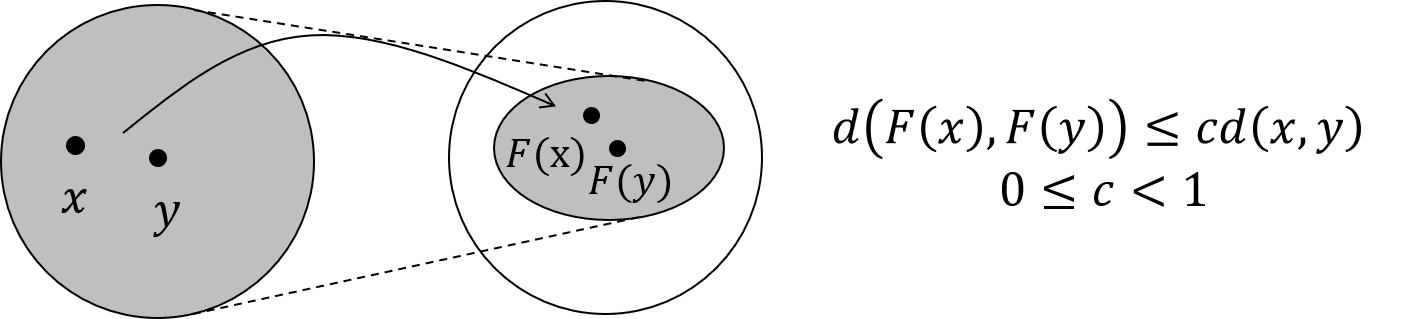### 具体实现

$𝐡_𝑣^{𝑡+1}=𝑓(𝐱_𝑣,𝐱_𝑐𝑜[𝑣] ,𝐡^t_𝑛𝑒[𝑣] ,𝐱_𝑛𝑒[𝑣])$

$=\sum_{𝑢∈𝑛𝑒[𝑣]} FNN([𝐱_𝑣;𝐱_{(𝑢,𝑣)};𝐡_𝑢^𝑡;𝐱_𝑢])$

$||F(x)-F(y)||{\leq}c||x-y||, 0\ {\leq}c<1$

$\frac{||F(x)-F(y)||}{||x-y||}{\leq}c$

$\frac{||F(x)-F(x-{\Delta}x)||}{||{\Delta}x||}{\leq}c$

$||F'(x)||=||\frac{{\partial}F(x)}{{\partial}x}||{\leq}c$

$J = Loss + \lambda \cdot \max({\frac{||{\partial}FNN||}{||{\partial}\mathbf{h}||}}−c,0), c\in(0,1)$

### 模型学习

$L𝑜𝑠𝑠=∑_{𝑖=1}^𝑝{(𝐭_𝑖−𝐨_𝑖)}$

1. 调用 $$f$$ 若干次，比如 $$T_n$$次，直到 $$\mathbf{h}^{T_{n}}_v$$ 收敛。
2. 此时每个结点的隐藏状态接近不动点的解。
3. 对于有监督信号的结点，将其隐藏状态通过 $$g$$ 得到输出，进而算出模型的损失。

### GNN与RNN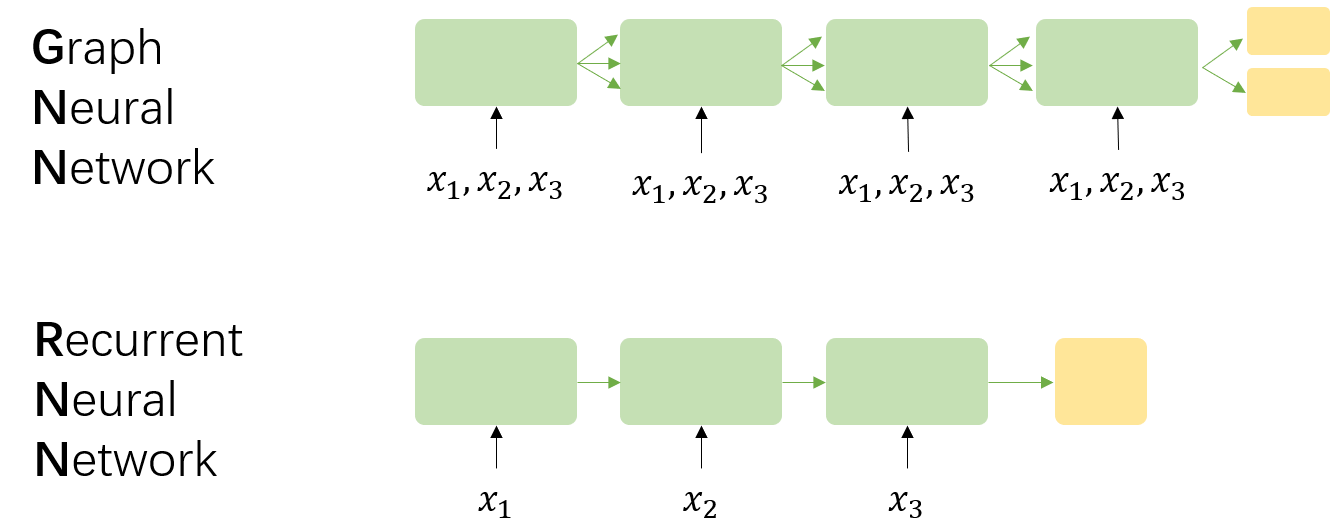• GNN的基础理论是不动点理论，这就意味着GNN沿时间展开的长度是动态的，是根据收敛条件确定的，而RNN沿时间展开的长度就等于序列本身的长度
• GNN每次时间步的输入都是所有结点 $$v$$ 的特征，而RNN每次时间步的输入是该时刻对应的输入。同时，时间步之间的信息流也不相同，前者由边决定，后者则由序列的读入顺序决定。
• GNN采用 AP 算法反向传播优化，而RNN使用BPTT(Back Propogation Through Time)优化。前者对收敛性有要求，而后者对收敛性是没有要求的。
• GNN循环调用 $$f$$ 的目标是得到每个结点稳定的隐藏状态，所以只有在隐藏状态收敛后才能输出；而RNN的每个时间步上都可以输出，比如语言模型。

### GNN的局限

• GNN只将边作为一种传播手段，但并未区分不同边的功能。虽然我们可以在特征构造阶段($$\mathbf{x}_{(u,v)}$$)为不同类型的边赋予不同的特征，但相比于其他输入，边对结点隐藏状态的影响实在有限。并且GNN没有为边设置独立的可学习参数，也就意味着无法通过模型学习到边的某些特性。
• 如果把GNN应用在图表示的场景中，使用不动点理论并不合适。这主要是因为基于不动点的收敛会导致结点之间的隐藏状态间存在较多信息共享，从而导致结点的状态太过光滑(Over Smooth)，并且属于结点自身的特征信息匮乏(Less Informative)。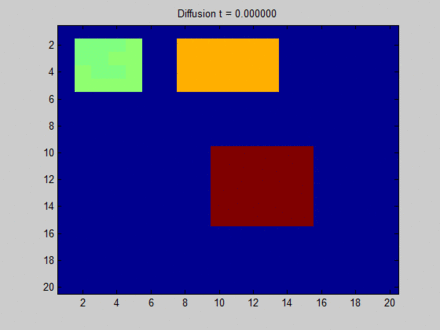## 门控图神经网络(Gated Graph Neural Network)

### 状态更新

$\mathbf{h}^{t+1}_v=\text{GRU}(\mathbf{h}^{t}_v,\sum_{𝑢∈𝑛𝑒[𝑣]} \mathbf{W}_{edge}𝐡_𝑢^𝑡)$

• 用结点特征初始化各个结点的(部分)隐藏状态。
• 对整张图，按照上述状态更新公式固定迭代若干步。
• 对部分有监督信号的结点求得模型损失，利用BPTT算法反向传播求得$$\mathbf{W}_{edge}$$和GRU参数的梯度。

### 实例1:到达判断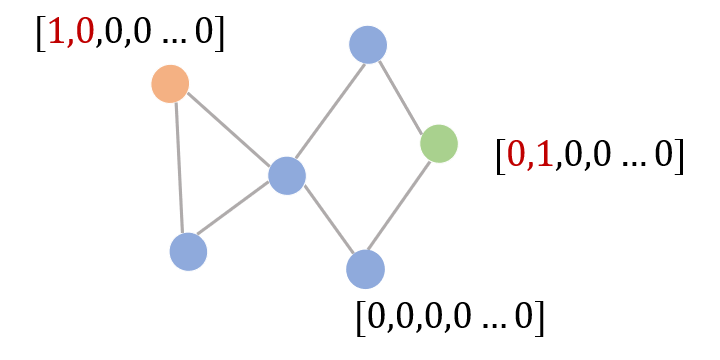### 实例2:语义解析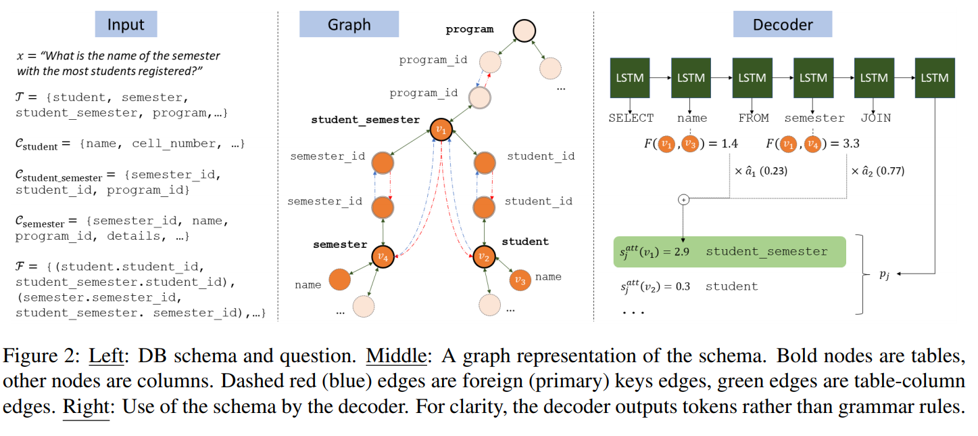3. 在解码阶段(Decoding)，如果输出的是表格中的Header/Table这类词，就用输出的向量与表格所有Header/Table的隐藏状态算一个分数，这个分数由$$F$$提供的。$$F$$实际上是一个全连接层，它的输出实际上是SQL单词与表格中各个Header/Table的联系程度。为了让SQL的每个输出都与历史的信息一致，每次输出时都用之前输出的Header/Table对候选集中的Header/Table打分，这样就利用到了多表的信息。

Model Acc Single Multi
No GNN 34.9% 52.3% 14.6%
GNN 40.7% 52.2% 26.8%

### GNN与GGNN

GGNN目前得到了广泛的应用，相比于GNN，其最大的区别在于不再以不动点理论为基础，虽然这意味着不再需要迭代收敛，但同时它也意味着GGNN的初始化很重要。从笔者阅读过的文献来看，GNN后的大部分工作都转向了将GNN向传统的RNN/CNN靠拢，可能的一大好处是这样可以不断吸收来自这两个研究领域的改进。但基于原始GNN的基于不动点理论的工作非常少，至少在笔者看文献综述的时候并未发现很相关的工作。

• 循环神经网络的好处在于能够处理任意长的序列，但它的计算必须是串行计算若干个时间步，时间开销不可忽略。所以，上面两种基于循环的图神经网络在更新隐藏状态时不太高效。如果借鉴深度学习中堆叠多层的成功经验，我们有足够的理由相信，多层图神经网络能达到同样的效果。
• 基于循环的图神经网络每次迭代时都共享同样的参数，而多层神经网络每一层的参数不同，可以看成是一个层次化特征抽取(Hierarchical Feature Extraction)的方法。

## 参考文献

. A Comprehensive Survey on Graph Neural Networks, https://arxiv.org/abs/1901.00596

. The graph neural network model, https://persagen.com/files/misc/scarselli2009graph.pdf

. Spectral networks and locally connected networks on graphs, https://arxiv.org/abs/1312.6203

. Distributed Representations of Words and Phrases and their Compositionality, http://papers.nips.cc/paper/5021-distributed-representations-of-words-andphrases

. DeepWalk: Online Learning of Social Representations, https://arxiv.org/abs/1403.6652

. Translating Embeddings for Modeling Multi-relational Data, https://papers.nips.cc/paper/5071-translating-embeddings-for-modeling-multi-relational-data

. Deep Learning on Graphs: A Survey, https://arxiv.org/abs/1812.04202

. 如何理解Graph Convolutional Network（GCN）? https://www.zhihu.com/question/54504471

. Almeida–Pineda recurrent backpropagation, https://www.wikiwand.com/en/Almeida–Pineda_recurrent_backpropagation

. Gated graph sequence neural networks, https://arxiv.org/abs/1511.05493

. Representing Schema Structure with Graph Neural Networks for Text-to-SQL Parsing, https://arxiv.org/abs/1905.06241

. Spider1.0 Yale Semantic Parsing and Text-to-SQL Challenge, https://yale-lily.github.io/spider

. Graph Neural Networks: A Review of Methods and Applications, https://arxiv.org/pdf/1812.08434

posted @ 2019-06-09 12:26  SivilTaram  阅读(105637)  评论(59编辑  收藏  举报